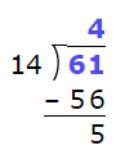Middle School Math, Warm-Up #3
Various Calculations
What is the easiest way to multiply by 10, 100, ...?
1 point
The decimal number 12.56 is multiplied by 100. Select the statement that is true.
1 point
The decimal number 12.56 is divided by 100. Select the statement that is true.
1 point
What is the best way to multiply 80x90
1 point
The expression 7.08 ÷ 100 is given. The result of the division is
1 point
When it comes to Division, what is a Remainder?
1 point
The expression 61 ÷ 14 is given. Check all statements that are true.
1 point
Does the following diagram depict the 61 ÷ 14 division correctly?
1 pointWhat is the remainder of this division?
1 pointWhich of the following relationships are correct based on the given diagram? Check all that apply.
1 pointWhat is the quotient and the remainder of the 46 ÷ 11 division?
2 points
4
2
46
11
quotient
remainder
What is the quotient and the remainder of the 39 ÷ 10 division?
2 points
39
10
9
3
quotient
remainder
Operations that move the decimal point to the right make the number
1 point
Operations that move the decimal point to the left make the number
1 point
Adding 2 trailing zeros to the rightmost position of a decimal number, for example 5.708 has the following effect
1 point
Adding 2 trailing zeros to the leftmost position of a decimal number, for example 5.708 has the following effect (check all that apply)
1 point
Inserting 2 zeros immediately to the right side of the decimal point of 5.708 has the following effect
1 point
Inserting 2 zeros immediately to the left side of the decimal point of 5.708 has the following effect
1 point